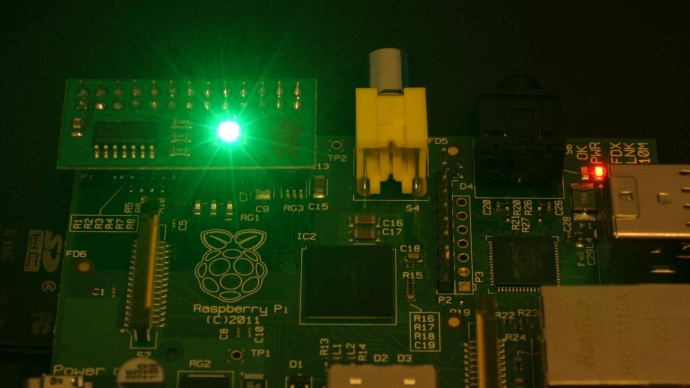# TimeMe - Time a command and compare with a target

This is an old driver based LedBorg script
We recommend using the new driver free based scripts for LedBorg.
The new driver free examples can be found here, the installation can be found here.

Ever wondered how you can time a command on your Raspberry Pi, or better yet how to make your LedBorg show you how long it took?
Well now you can with TimeMe.py, it calculates the time a command takes, compares it with your target and shows you the results visually:0%to22% 23%to44% 45%to67% 68%to89% 90%to110%target :) 111%to132% 133%to155% 156%to177% 178%ormore

Here's the code, you can download the TimeMe script file as text here
Save the text file on your pi as TimeMe.py
Make the script executable using
`chmod +x TimeMe.py`
and run using
`./TimeMe.py time_in_seconds command`
e.g.
`./TimeMe.py 0.15 du ~/`

```#!/usr/bin/env python
# coding: latin-1

# Import library functions we need
import time
import sys
import os

# Function to set the LedBorg colour
def SetColour(colour):
LedBorg=open('/dev/ledborg','w')
LedBorg.write(colour)
LedBorg.close()

# Function to format a number of seconds into a human readable form
def FormatSeconds(seconds):
if seconds >= 60.0:
minutes = int(seconds / 60.0)
seconds -= minutes * 60.0
text = '%d:%06.3f' % (minutes, seconds)
elif seconds < 1.0:
text = '%03.0f ms' % (seconds * 1000.0)
else:
text = '%02.3f s' % (seconds)
return text

# Set up our chart, from 0% of target to 200% of target
colours = ['002', '012', '022', '021', '020', '120', '220', '210', '200']
colourBusy = '101'

# Get user input
if len(sys.argv) > 2:
try:
target = float(sys.argv)
printUsage = False
except ValueError:
# Not a valid number
print '"%s" is not a number!' % (sys.argv)
printUsage = True
else:
printUsage = True

if printUsage:
print 'Usage: %s target_in_seconds command' % (sys.argv)
else:
# Form the command from parts
command = ''
for part in sys.argv[2:]:
command += part + ' '
# Start the timer
SetColour(colourBusy)
before = time.time()
# Run the command
os.system(command)
# Stop the timer
after = time.time()
lapsed = after - before
# Calculate the time statistics
if target == 0:
ratio = 0
else:
ratio = lapsed / target
percentage = ratio * 100.0
# Work out which colour to show
index = (ratio / 2.0) * len(colours)
if index >= len(colours):
index = len(colours) - 1
else:
index = int(index)
# Display the statistics
SetColour(colours[index])
print ''
print 'Command: ' + command
print 'Target: ' + FormatSeconds(target)
print 'Actual: ' + FormatSeconds(lapsed)
print 'Percentage: %03.2f%%' % (percentage)
```